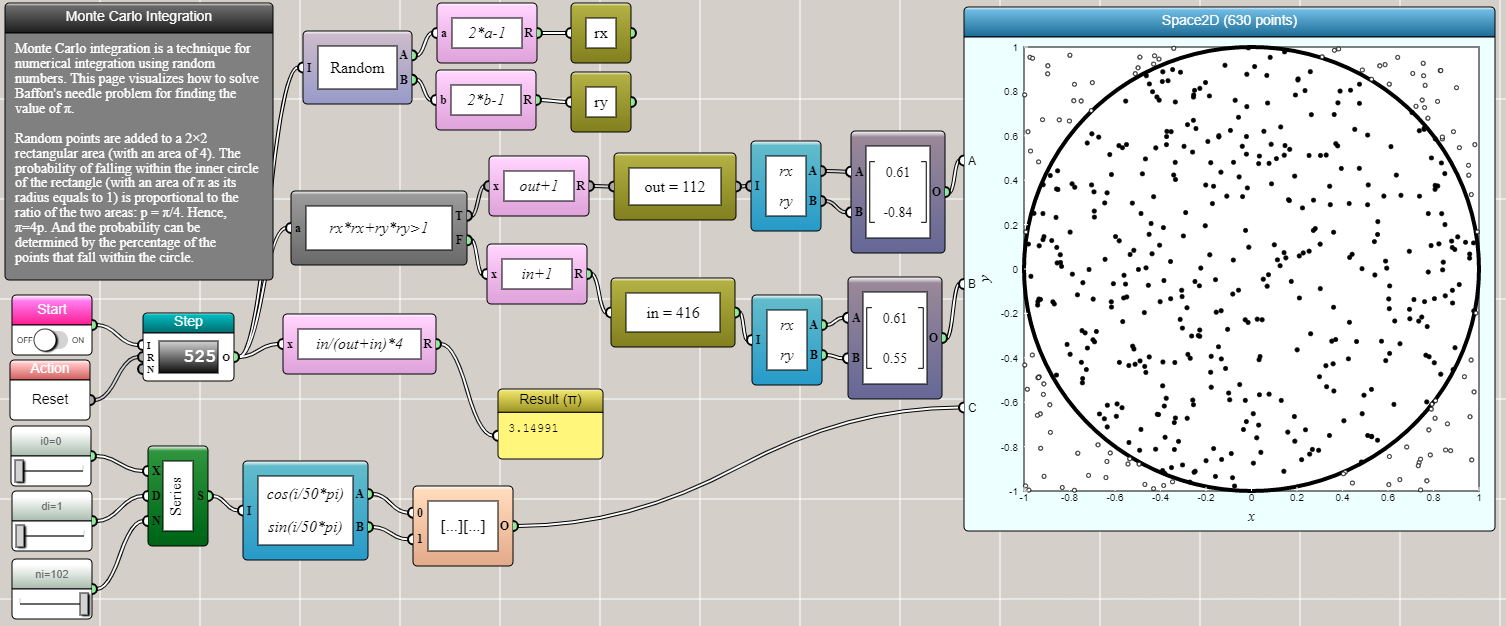#### Monte Carlo Methods

By Charles Xie

Monte Carlo methods are a class of algorithms that uses random sampling to obtain numerical approximations to problems that might be deterministic in nature. They are most useful when it is difficult or impossible to use other approaches.

##### Monte Carlo integration

Buffon's needle problem is an example of using a Monte Carlo method to approximate the value of π. The following screenshot shows a variation of the problem: If we throw dots onto a 1×1 square, what is the probability of them falling within the unit circle enclosed in the square?